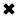# Reactive Power Explained#### Reactive power is measured in kilovolt ampere reactive (kVAR) or MVAR.

Reactive Power is the power which flows back and froth that mean it moves in both the direction in the circuit or react upon itself, is called Reactive Power. Reactive power is measured in kilovolt ampere reactive (kVAR) or MVAR.

Reactive power is a type of power that does no real work and is generally associated with reactive elements (inductors and capacitors). For example, the inductance of a load such as a motor causes the load current to lag behind the voltage. Power appearing across the inductance sloshes back and forth between the inductance itself and the power sources producing no net work For this reason it is called imaginary or reactive power since no power is dissipated or expended. It is expressed in units of volt-ampere-reactive or var. In the sinusoidal case, the reactive power is simply defined aswhich is the portion of power in quadrature with the active power and demonstrates the relationship between P, Q, and S in sinusoidal condition.

There is some disagreement among harmonics analysts on how to define Q in the presence of harmonic distortion. If it were not for the fact that many utilities, which produce magnetic fields in transmission lines, measure Q and compute demand billing from the power factor computed by Q, it might be a moot point. It is more important to determine P and S; P defines how much active power is being consumed while S defines the capacity of the power system required to deliver P. Q is not actually very useful by itself. However, Q1 the traditional reactive power component at fundamental frequency, may be used to size shunt capacitors.Relationship between P, Q, and S in a sinusoidal condition

The reactive power, when distortion is present, has another interesting peculiarity. In fact, it may not be appropriate to call it reactive power. The concept of var flow in the power system is deeply ingrained in the minds of most power engineers. What many do not realize is that this concept is valid only in the sinusoidal steady state. When distortion is present (as in the case of induction motors), the component of S that remains after P is taken out, is not conserved—that is, it does not sum to zero at a node. Power quantities are presumed to flow around the system in a conservative manner.

This does not imply that P is not conserved or that current is not conserved because the conservation of energyktnd Kirchoff's current laws are still applicable for any waveform. The reactive components actually sum in quadrature (square root of the sum of the squares). This has prompted some analysts to propose that Q be used to denote the reactive components that are conserved and introduce a new quantity for the components that are not. Many call this quantity D, for distortion power or, simply, distortion voltamperes. It has units of voltamperes, but it may not be strictly appropriate to refer to this quantity as power, because it does not flow through the system as power is assumed to do. In this concept, Q consists of the sum of the traditional reactive power values at each frequency. D represents all cross products of voltage and current at different frequencies, which yield no average power. P, Q, D, and S are related as follows, using the definitions for S and P above as a starting point:Some prefer to use a three-dimensional vector chart to demonstrate Reactive Power relationships from the components. P and Q contribute the traditional sinusoidal components to S while represents the additional contribution to the apparent power by the harmonics.

There are many factors to consider when determining and measuring reactive power in an ac circuit in any power plant:

• Real power
• Voltage level
• Purely resistive true power
• Active reactive and apparent power
• Active and reactive powers
• How to absorb reactive power

##### Basic Electricity Handbook, Vol. 1• FULLY ILLUSTRATED
• GREAT FOR SCHOOL PROJECTS
• GREAT PRICE: \$5.99
• ...

This 100+ page e-book is a great guide for those who have a basic interest in the field of electricity. This well-illustrated e-book, coupled with some basic knowledge of electricity, will give you a broad theoretical background in this fundamental subject.

CONTENTS
• FUNDAMENTALS OF ELECTRICITY
• HOW DOES ELECTRICITY WORK?
• HOW IS ELECTRICITY GENERATED?
• WHERE DOES ELECTRICITY COME FROM?
• BASIC HOME WIRING
• ELECTRICITY FOR STUDENTS
• ENERGY SAVING TIPS

##### TRAINING EF COURSES

Content Community Connection
Top